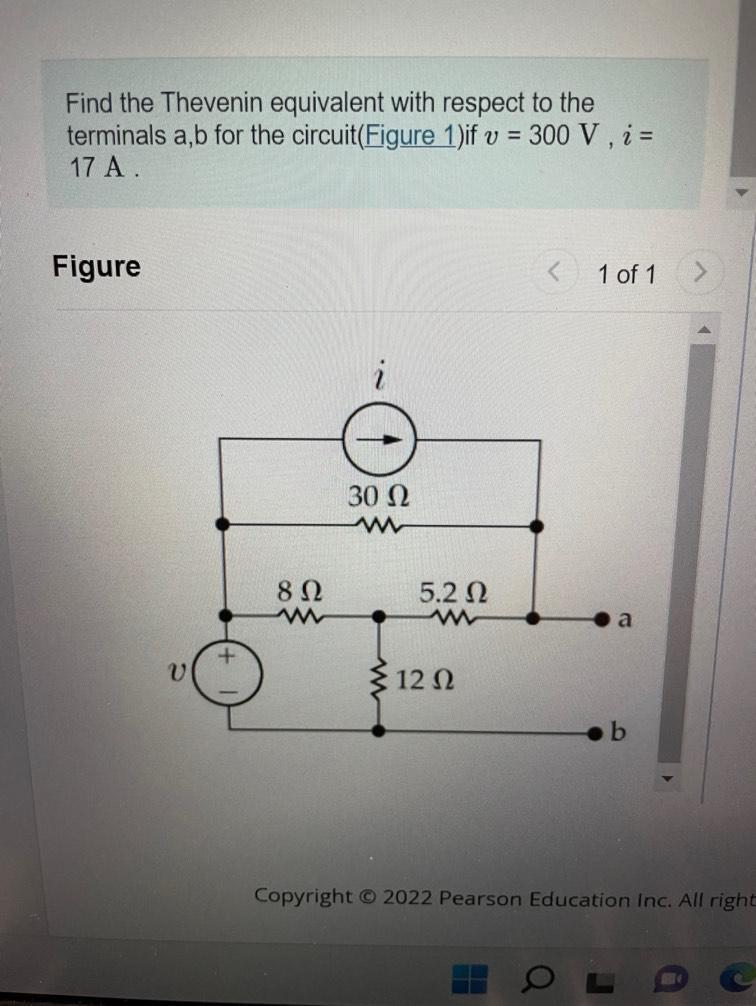Question:

# Find the Thevenin equivalent with respect to the terminals a, b for the circuit(Figure 1)if v = 300 V, i = 17 A Figure 1 of 1 iFind the Thevenin equivalent with respect to the terminals a, b for the circuit(Figure 1)if v = 300 V, i = 17 A Figure 1 of 1 i 30 22 812 5.212 ww 12 12 b Copyright © 2022 Pearson Education Inc. All right